Maths for Chemists - Tips for teaching maths skills to our future chemists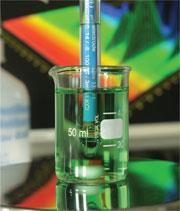Source: Shutterstock

### Teaching approaches

In secondary education and first year university student’s chemistry textbooks, the chapters regarding calculations of pH for either a Brønsted-Lowry weak acid or weak base solution are taught as separate topics.1–3

For example, students may be asked to calculate the pH of a 0.1 M ammonium chloride solution and in another problem calculate the pH of a 0.1 M sodium benzoate solution. In one textbook2 there is a limited discussion, without calculations, about the pH of a salt solution containing a Brønsted-Lowry weak acid and weak base in which the Ka and Kb are equivalent,eg ammonium acetate.

In essence, the pH is 7 because the acid and base characteristics of the reactants are equal to each other, for the conjugate acid and base Ka = Kb, and the production of hydroxonium and hydroxide ions is equivalent, making water via a neutralisation reaction (Equations 1–4).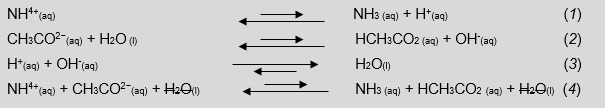This is a three step technique, which is fairly accurate, for estimating the pH of a solution where Ka and Kb are unequal. Ammonium salts were selected due to their high solubility. Transition metal ions with Brønsted-Lowry bases are insoluble to slightly soluble and there is the issue of production of transition metal hydroxide precipitates.

Ammonium oxalate is used as an example in this discussion; the Ka is 5.6x10-10 M while the Kb is 6.5 x10-11 M.

Looking at Equations 5–7, we can point out to students that calculating a final pH of a solution containing a Brønsted-Lowry acid and base with unequal equilibrium constants is virtually impossible. This is because there is a disproportionate production of hydroxonium or hydroxide ions that will react to produce water, leave an excess unknown amount of either H+(aq) or OH-(aq) and, in turn, shift interdependent equilibriums.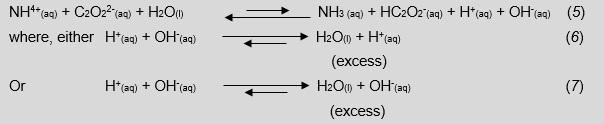### Estimating pH

The following three step technique for estimating pH is empirically derived and requires the following constraints:

1. The pKb of the anion is restricted to 8 ±2 when using ammonium ions.
2. The anion can neither have any acidic hydrogen atoms nor be a zwitterion.

Our research demonstrated that pH readings for ammonium salt solutions with concentrations less than 0.4 M varied with the slightest temperature change from 25°C or with minimal exposure to atmospheric gases, namely carbon dioxide. We found that 0.4 M solutions at room temperature had constant pH readings after a long duration of exposure to atmospheric gases and were in compliance with readings of 0.1 M solutions at 25°C and having a nitrogen blanket. Therefore, our final research was conduction at 0.4 M, which is also a good concentration for student experimentation.

### Practical notes

1. Distilled water was degassed of atmospheric gases using helium. A nitrogen blanked was also employed prior to making any solution under 0.4 M.
2. When ammonium salts needed to be made, then a mixture of ammonium chloride and the sodium salt of a weak acid was used.
3. When a sodium salt was not readily available, the weak acid was stoichiometrically titrated with aqueous sodium hydroxide.

### Sample calculation using 0.4 M ammonium oxalate, (NH4)2C2O4

#### Step one

Calculate a pH value for ammonium ions by following the customary method for computing acidity of a weak acid solution while assuming ammonia’s equilibrium is unaffected by the presence of oxalate ions:Or, using a general equation with logs, we may write: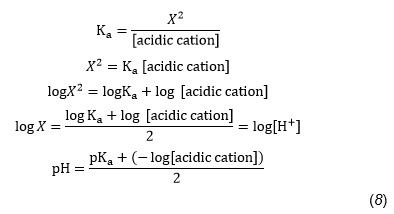and returning to our example,### Step two

Calculate a pOH value for oxalate ionsby using Equation 9, which is a modification of Equation 8,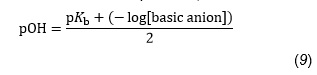we may now write:#### Step three

Add the pH and pOH values and compare the sum to that of pKw at 25°C.

The sum, in this example, does not add to 14.00 and the difference (14.00-9.96) is 4.04. In this technique, add one half of the difference to the estimated pH value and the other half to that of pOH – see Table 1.

Table 1 – Calculations for adjusting pH and pOH values
Estimated pHEstimated pOHSumDifferencefrom 14.00

4.67

5.29

9.96

4.04

Half the difference

2.02

2.02

---

---

Sum of values

6.69

7.31

14.00

0

The estimated pH of the solution is 6.69 while the measured value is 6.44. Based upon the data in Table 2, the uncertainty for estimated pH has been determined to be ±0.3 pH units. Students would report their answer as 6.6 ±0.3. Table 3 lists truncated data for estimated and measured pH values. Data are truncated due to the impact of rounding logs.

Table 2 - Ammonium salts and pH values. The 0.4 M solutions are at 25°C
Ammonium saltsFormulapKa of anion4,5Calculated pKb of anionEstimated pHMeasured pH±Δ pH

Acetate

NH4 C2 H3 O2

4.756

9.244

7.00

6.98

0.02

Benzoate

NH4 C7 H5 O2

4.204

9.796

6.87

6.62

0.25

Citrate

(NH4)2 C6 H5 O7

4.76

9.24

6.92

7.22

0.30

Malonate

(NH4)2 C3 H2 O4

5.7

8.3

7.1

7.1

0

1-Naphthyacetate

NH4 C12 H10 O2

4.236

9.764

6.88

7.00

0.12

Oxalate

(NH4)2 C2 O4

3.81

10.19

6.69

6.44

0.25

Sufite

(NH4)2 SO3

7.2

6.8

7.5

7.8

0.3

DL-Tartrate

(NH4)2 C4 H4 O6

4.37

9.63

6.83

6.84

0.01

P-Toluate

NH4 C8 H7 O2

4.37

9.63

6.83

7.08

0.25

Table 3 - Truncated pH values from Table 2
Ammonium saltsTruncated estimated pH valuesTruncated measured pH

Acetate

7.0

6.9

Benzoate

6.8

6.6

Citrate

6.9

7.2

Malonate

7.1

7.1

1-Naphthyacetate

6.8

7.0

Oxolate

6.6

6.4

Sufite

7.5

7.8

DL-Tartrate

6.8

6.8

P-Toluate

6.8

7.1

### Conclusion

The application of this simple technique is limited in application in a manner reminiscent to that of the Ideal Gas Law. Nevertheless, this approach to pH calculations is an introduction for students to the quite complex nature of equilibrium between Brønsted-Lowry acids and bases and also allows for a discussion into an area not taught in secondary education and first year university chemistry.

Manus Monroe is a lecturer in chemistry at Sonoma State University, California, US.